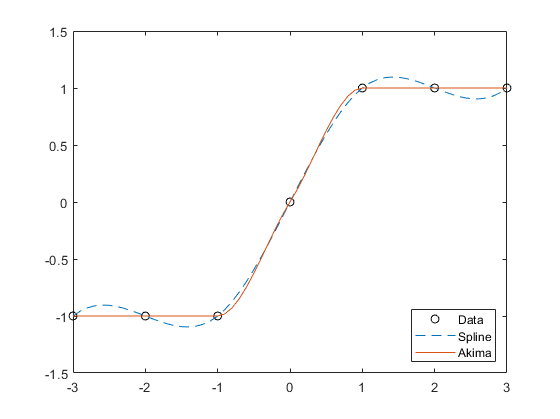# interp1

## 语法

``vq = interp1(x,v,xq)``
``vq = interp1(x,v,xq,method)``
``vq = interp1(x,v,xq,method,extrapolation)``
``vq = interp1(v,xq)``
``vq = interp1(v,xq,method)``
``vq = interp1(v,xq,method,extrapolation)``
``pp = interp1(x,v,method,'pp')``

## 说明

``vq = interp1(x,v,xq)` 使用线性插值返回一维函数在特定查询点的插入值。向量 `x` 包含样本点，`v` 包含对应值 v(x)。向量 `xq` 包含查询点的坐标。如果您有多个在同一点坐标采样的数据集，则可以将 `v` 以数组的形式进行传递。数组 `v` 的每一列都包含一组不同的一维样本值。`

``vq = interp1(x,v,xq,method)` 指定备选插值方法：`'linear'`、`'nearest'`、`'next'`、`'previous'`、`'pchip'`、`'cubic'`、`'v5cubic'`、`'makima'` 或 `'spline'`。默认方法为 `'linear'`。`

``vq = interp1(x,v,xq,method,extrapolation)` 用于指定外插策略，来计算落在 `x` 域范围外的点。如果希望使用 `method` 算法进行外插，可将 `extrapolation` 设置为 `'extrap'`。您也可以指定一个标量值，这种情况下，`interp1` 将为所有落在 `x` 域范围外的点返回该标量值。`

````vq = interp1(v,xq)` 返回插入的值，并假定一个样本点坐标默认集。默认点是从 `1` 到 `n` 的数字序列，其中 `n` 取决于 `v` 的形状： 当 v 是向量时，默认点是 `1:length(v)`。当 v 是数组时，默认点是 `1:size(v,1)`。 如果您不在意点之间的绝对距离，则可使用此语法。```
``vq = interp1(v,xq,method)` 指定备选插值方法中的任意一种，并使用默认样本点。`
``vq = interp1(v,xq,method,extrapolation)` 指定外插策略，并使用默认样本点。`
````pp = interp1(x,v,method,'pp')` 使用 `method` 算法返回分段多项式形式的 v(x)。 注意不建议使用该语法。请改用 `griddedInterpolant`。 ```

## 示例

```x = 0:pi/4:2*pi; v = sin(x);```

`xq = 0:pi/16:2*pi;`

```figure vq1 = interp1(x,v,xq); plot(x,v,'o',xq,vq1,':.'); xlim([0 2*pi]); title('(Default) Linear Interpolation');``````figure vq2 = interp1(x,v,xq,'spline'); plot(x,v,'o',xq,vq2,':.'); xlim([0 2*pi]); title('Spline Interpolation');````v = [0 1.41 2 1.41 0 -1.41 -2 -1.41 0];`

`xq = 1.5:8.5;`

`vq = interp1(v,xq);`

```figure plot((1:9),v,'o',xq,vq,'*'); legend('v','vq');````x = 1:10;`

`v = (5*x)+(x.^2*1i);`

`xq = 1:0.25:10;`

`vq = interp1(x,v,xq);`

```figure plot(x,real(v),'*r',xq,real(vq),'-r'); hold on plot(x,imag(v),'*b',xq,imag(vq),'-b');``````x = (datetime(2016,1,1):hours(4):datetime(2016,1,2))'; x.Format = 'MMM dd, HH:mm'; T = [31 25 24 41 43 33 31]'; WeatherData = table(x,T,'VariableNames',{'Time','Temperature'})```
```WeatherData=7×2 table Time Temperature _____________ ___________ Jan 01, 00:00 31 Jan 01, 04:00 25 Jan 01, 08:00 24 Jan 01, 12:00 41 Jan 01, 16:00 43 Jan 01, 20:00 33 Jan 02, 00:00 31 ```
`plot(WeatherData.Time, WeatherData.Temperature, 'o')````xq = (datetime(2016,1,1):minutes(1):datetime(2016,1,2))'; V = interp1(WeatherData.Time, WeatherData.Temperature, xq, 'spline');```

```hold on plot(xq,V,'r')``````x = [1 2 3 4 5]; v = [12 16 31 10 6];```

`xq = [0 0.5 1.5 5.5 6];`

`vq1 = interp1(x,v,xq,'pchip')`
```vq1 = 1×5 19.3684 13.6316 13.2105 7.4800 12.5600 ```

`vq2 = interp1(x,v,xq,'linear')`
```vq2 = 1×5 NaN NaN 14 NaN NaN ```

`vq3 = interp1(x,v,xq,'linear','extrap')`
```vq3 = 1×5 8 10 14 4 2 ```

`'pchip'` 默认外插，但 `'linear'` 不会。

```x = [-3 -2 -1 0 1 2 3]; v = 3*x.^2;```

`xq = [-4 -2.5 -0.5 0.5 2.5 4];`

`vq = interp1(x,v,xq,'pchip',27)`
```vq = 1×6 27.0000 18.6562 0.9375 0.9375 18.6562 27.0000 ```

`x = (-5:5)';`

`x` 所定义的点处对三个不同的抛物线函数采样。

```v1 = x.^2; v2 = 2*x.^2 + 2; v3 = 3*x.^2 + 4;```

`v = [v1 v2 v3];`

`xq = -5:0.1:5;`

```vq = interp1(x,v,xq,'pchip'); figure plot(x,v,'o',xq,vq); h = gca; h.XTick = -5:5;```## 输入参数

• 如果 `v` 为向量，则 `length(x)` 必须等于 `length(v)`

• 如果 `v` 为数组，则 `length(x)` 必须等于 `size(v,1)`

`'linear'`

C0

• 需要至少 2 个点。

• 比最近邻点插值需要更多内存和计算时间。

`'nearest'`

• 需要至少 2 个点。

• 最低内存要求

• 最快计算时间

`'next'`

• 需要至少 2 个点。

• 内存要求和计算时间与 `'nearest'` 相同

`'previous'`

• 需要至少 2 个点。

• 内存要求和计算时间与 `'nearest'` 相同

`'pchip'`

C1

• 需要至少 4 个点。

• `'linear'` 需要更多内存和计算时间

`'cubic'`

C1

• 需要至少 3 个点。

• 点必须均匀间隔

• 对于不规则间隔的数据，此方法会回退到 `'spline'` 插值

• 内存要求和计算时间与 `'pchip'` 相似

`'v5cubic'`

`'cubic'` 相同。

C1

`'makima'`

C1

• 需要至少 2 个点。

• 产生的波动比 `'spline'` 小，但不像 `'pchip'` 那样急剧变平

• 计算成本高于 `'pchip'`，但通常低于 `'spline'`

• 内存要求与 `'spline'` 类似

`'spline'`

C2

• 需要至少 4 个点。

• `'pchip'` 需要更多内存和计算时间

• 如果希望 `interp1` 使用与内插所用相同的方法来计算落在域范围外的点，则指定 `'extrap'`

• 如果希望 `interp1` 为落在域范围外的点返回一个特定常量值，则指定一个标量值。

• 如果您指定 `'pchip'``'spline'``'makima'` 插值方法，则默认行为是 `'extrap'`

• 任何其他方法都会为落在域范围外的查询点默认返回 `NaN`

## 输出参数

v 的形状xq 的形状Vq 的大小示例

`size(xq) = [1 500]`
`size(vq) = [1 500]`

`size(xq)`如果 `size(v) = [1 100]`
`size(xq) = [50 30]`
`size(vq) = [50 30]`

`size(xq) = [1 500]`
`size(vq) = [500 3]`

`[size(xq,1),...,size(xq,n),... size(v,2),...,size(v,m)]`如果 `size(v) = [4 5 6]`
`size(xq) = [2 3 7]`
`size(vq) = [2 3 7 5 6]`

## 详细信息

### Akima 和样条插值

 中所述的一维插值 Akima 算法执行三次插值以生成具有连续一阶导数 (C1) 的分段多项式。该算法保持斜率，避免平台区的波动。每当有三个或更多连续共线点时，就会出现平台区，算法将这些点用一条直线相连。为了确保两个数据点之间的区域是平坦的，请在这两个点之间插入一个额外的数据点。Akima, Hiroshi. "A new method of interpolation and smooth curve fitting based on local procedures." Journal of the ACM (JACM) , 17.4, 1970, pp. 589-602.

 Akima, Hiroshi. "A method of bivariate interpolation and smooth surface fitting based on local procedures." Communications of the ACM , 17.1, 1974, pp. 18-20.